Question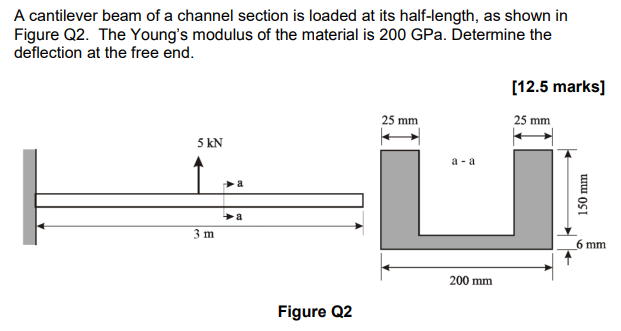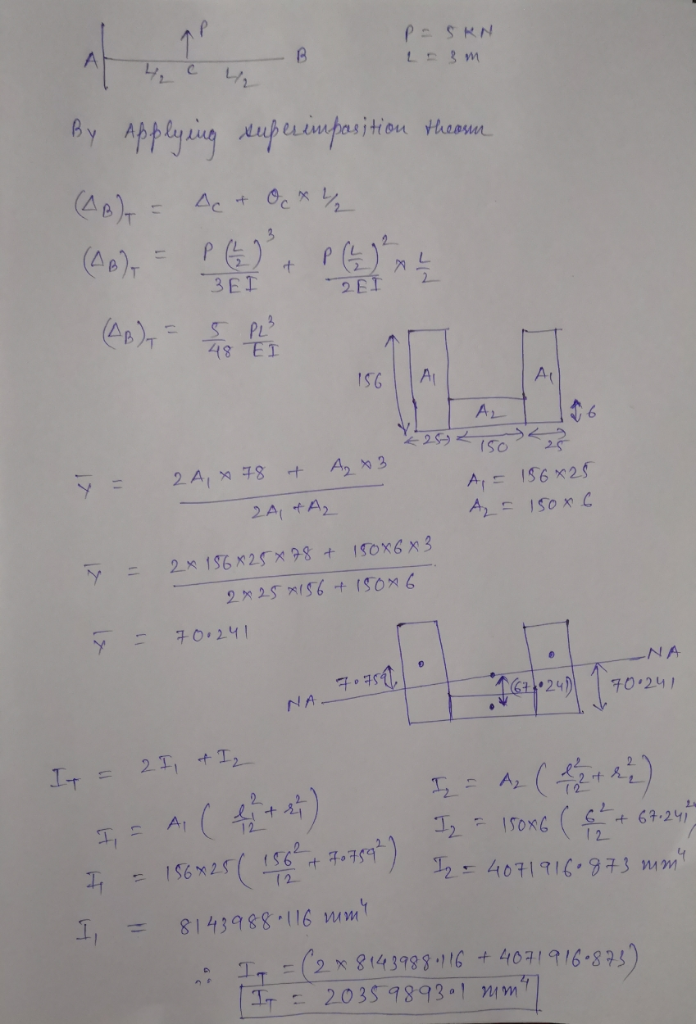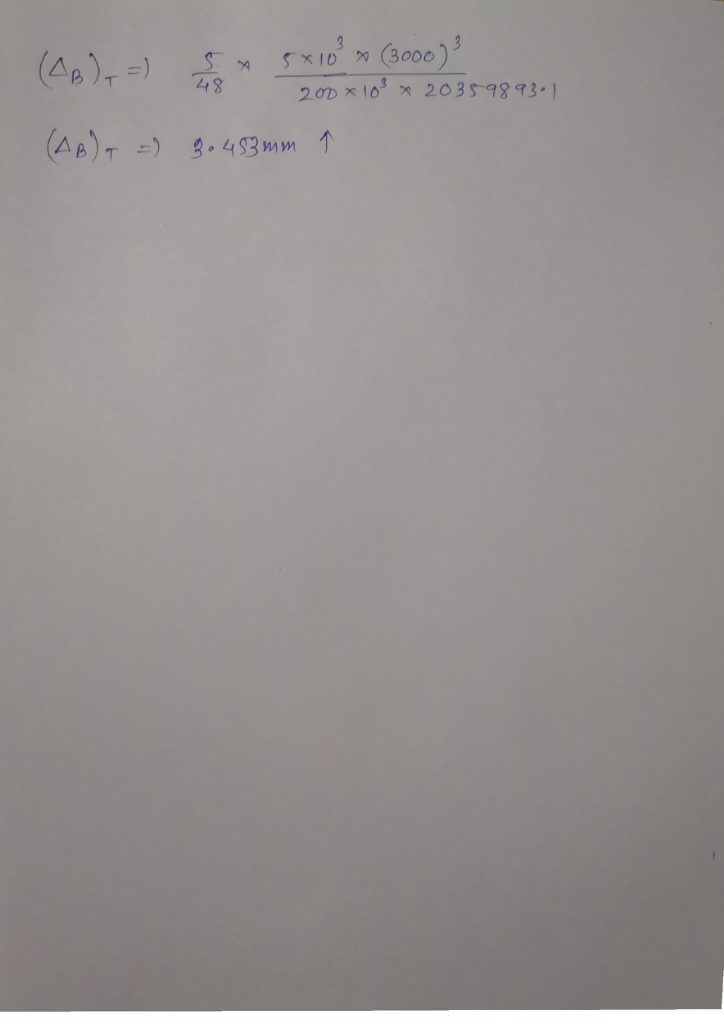#### Earn Coins

Coins can be redeemed for fabulous gifts.

Similar Homework Help Questions
• ### 8. The cantilever beam in Figure Q8 subjects to concentrated loading. The cross section geometry gives...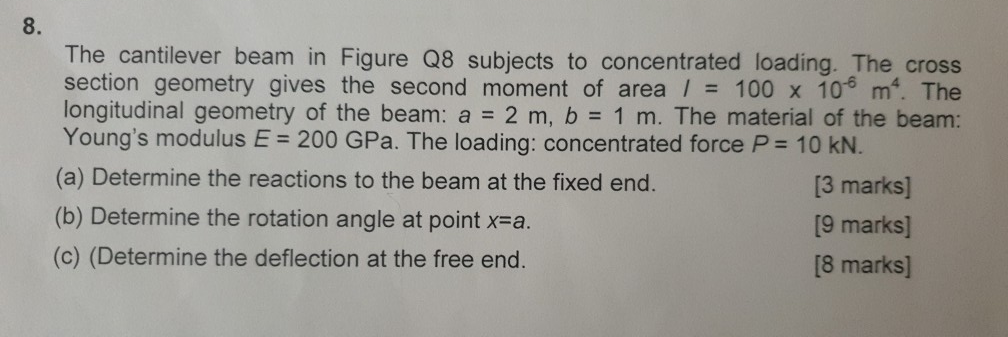8. The cantilever beam in Figure Q8 subjects to concentrated loading. The cross section geometry gives the second moment of area / 100 x 10 m. The longitudinal geometry of the beam: a 2 m, b 1 m. The material of the beam: Young's modulus E 200 GPa. The loading: concentrated force P 10 KN. (a) Determine the reactions to the beam at the fixed end. (b) Determine the rotation angle at point x-a (c) (Determine the deflection at the...

• ### Q1. For the cantilever beam and loading shown with circular section of 60 mm diameter and...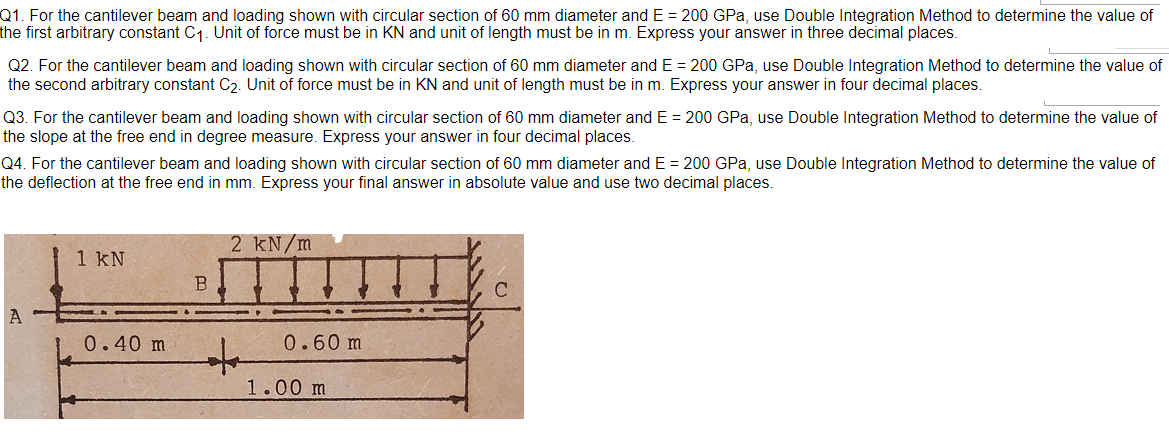Q1. For the cantilever beam and loading shown with circular section of 60 mm diameter and E = 200 GPa, use Double Integration Method to determine the value of the first arbitrary constant C1. Unit of force must be in KN and unit of length must be in m. Express your answer in three decimal places. Q2. For the cantilever beam and loading shown with circular section of 60 mm diameter and E = 200 GPa, use Double Integration Method...

• ### Q1 An elastic cantilever beam of varying cross section, as shown in Figure Q1(a), is subjected...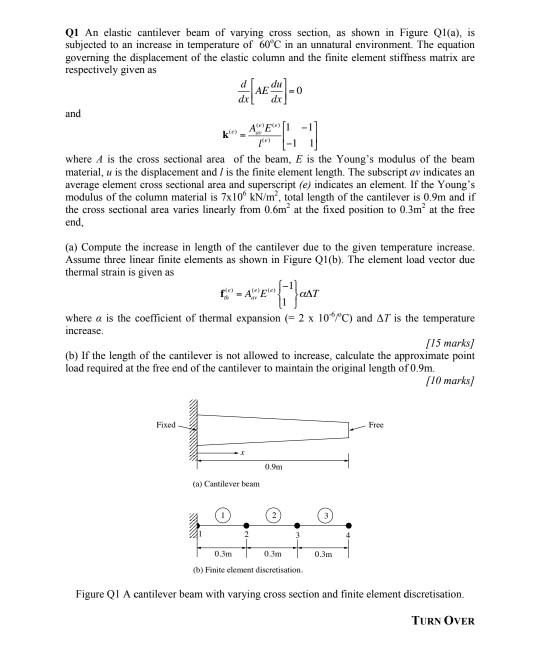Q1 An elastic cantilever beam of varying cross section, as shown in Figure Q1(a), is subjected to an increase in temperature of 60°C in an unnatural environment. The equation governing the displacement of the elastic column and the finite element stiffness matrix are respectively given as -O and ΑΕ) - where A is the cross sectional area of the beam, E is the Young's modulus of the beam material, u is the displacement and / is the finite element length....

• ### 4. Determine the slope and deflection at end point C of the cantilever beam shown in...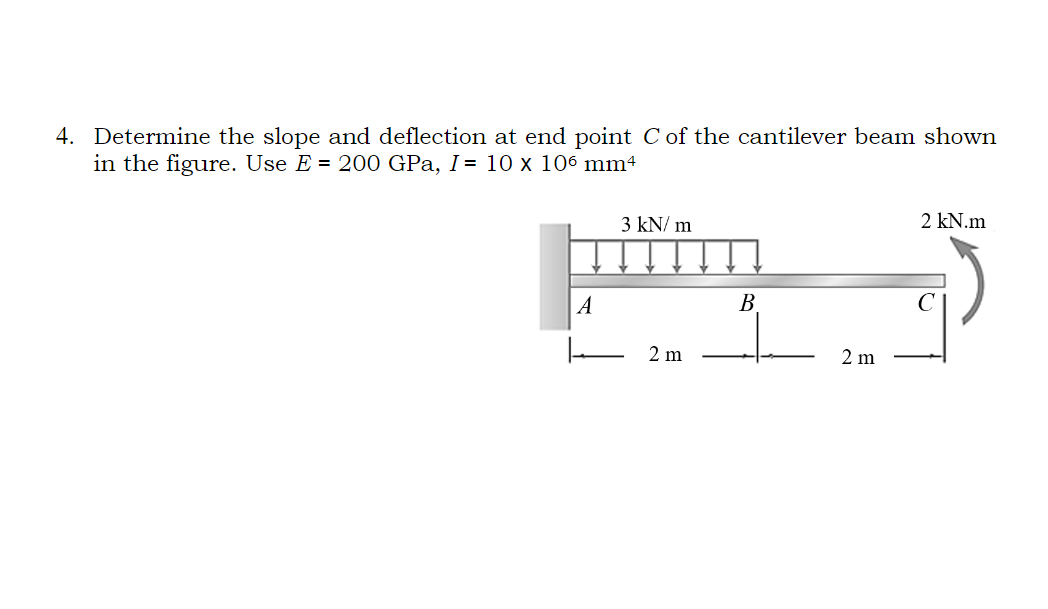4. Determine the slope and deflection at end point C of the cantilever beam shown in the figure. Use E = 200 GPa, I = 10 x 106 mm 3 kN/m 2 kN.m A B 2 m 2 m

• ### Strength of Material 3.7 A cantilever with a length of 1,5 m has a cross-section as...Strength of Material 3.7 A cantilever with a length of 1,5 m has a cross-section as shown in figure 3.16. Calculate: a) the magnitude of the allowable vertical load at the free end if the maximum allowable stress is 150 MPa, b) the deflection at the free end. E = 200 GPa. [2,97 kN; 13,57 mm) .8 12 112 80 ALERY Figure 3.16 A2 = 30 y los 69

• ### The cantilever beam AC with length L=5 m has elasticity modulus E= 172 GPa, cross-section with...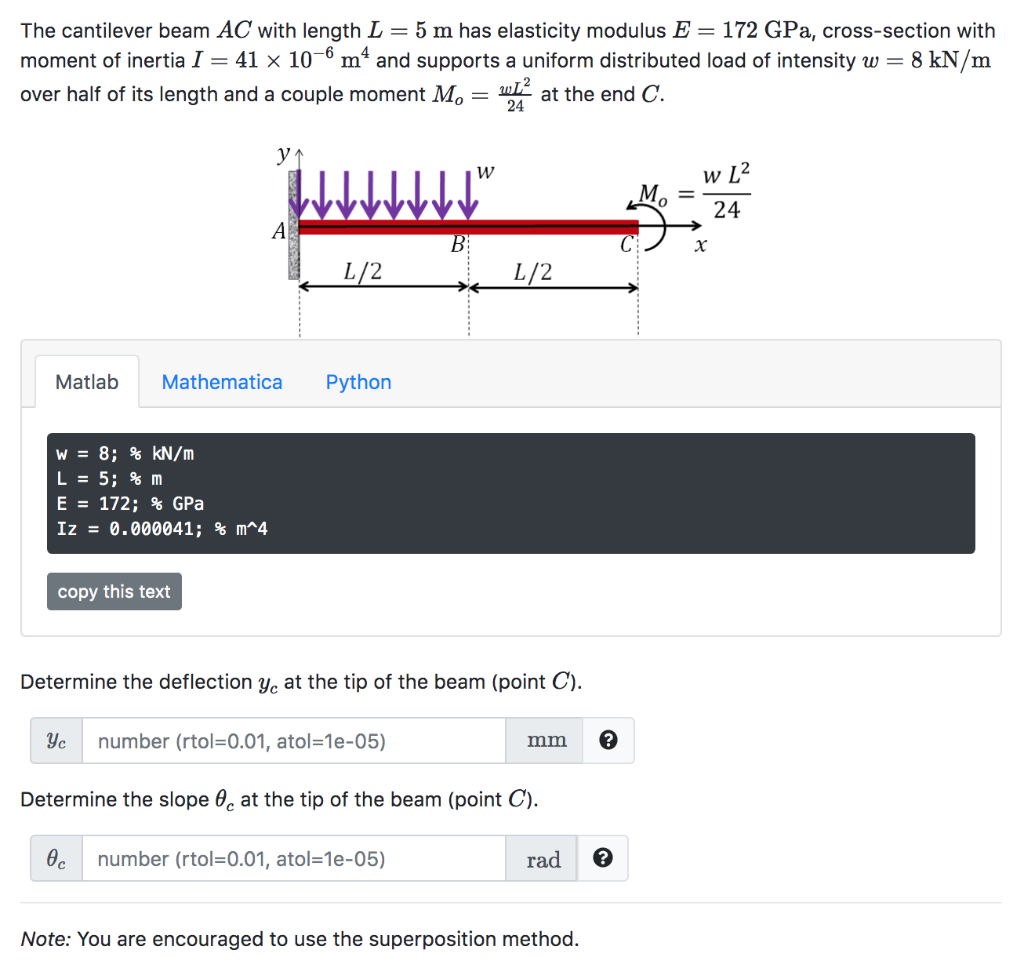The cantilever beam AC with length L=5 m has elasticity modulus E= 172 GPa, cross-section with moment of inertia I = 41 x 10-6 mº and supports a uniform distributed load of intensity w = 8 kN/m over half of its length and a couple moment Mo = win at the end C. W w L2 VV WWWV В x 1 1/2 1/2 C) . Matlab Mathematica Python W = 8; % kN/m L = 5;%m E = 172; %...

• ### For the cantilever beam shown in figure below, we have derived the deflection curve during the...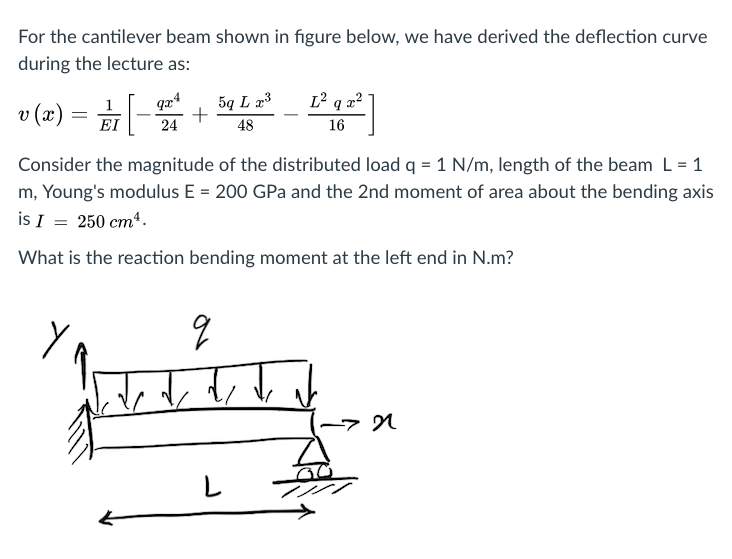For the cantilever beam shown in figure below, we have derived the deflection curve during the lecture as: r(z)-하-둬뿌 부] 48 Consider the magnitude of the distributed load q 1 N/m, length of the beam L 1 m, Young's modulus E-200 GPa and the 2nd moment of area about the bending axis is 1 = 250 cm". What is the reaction bending moment at the left end in N.m? Ya 2

• ### Q5. The cantilever beam, AC, is subjected to the load case shown in Figure 5. For the loading shown, do the following: [10 Marks] a) Calculate the magnitude and direction of the reactions at A b) Usi...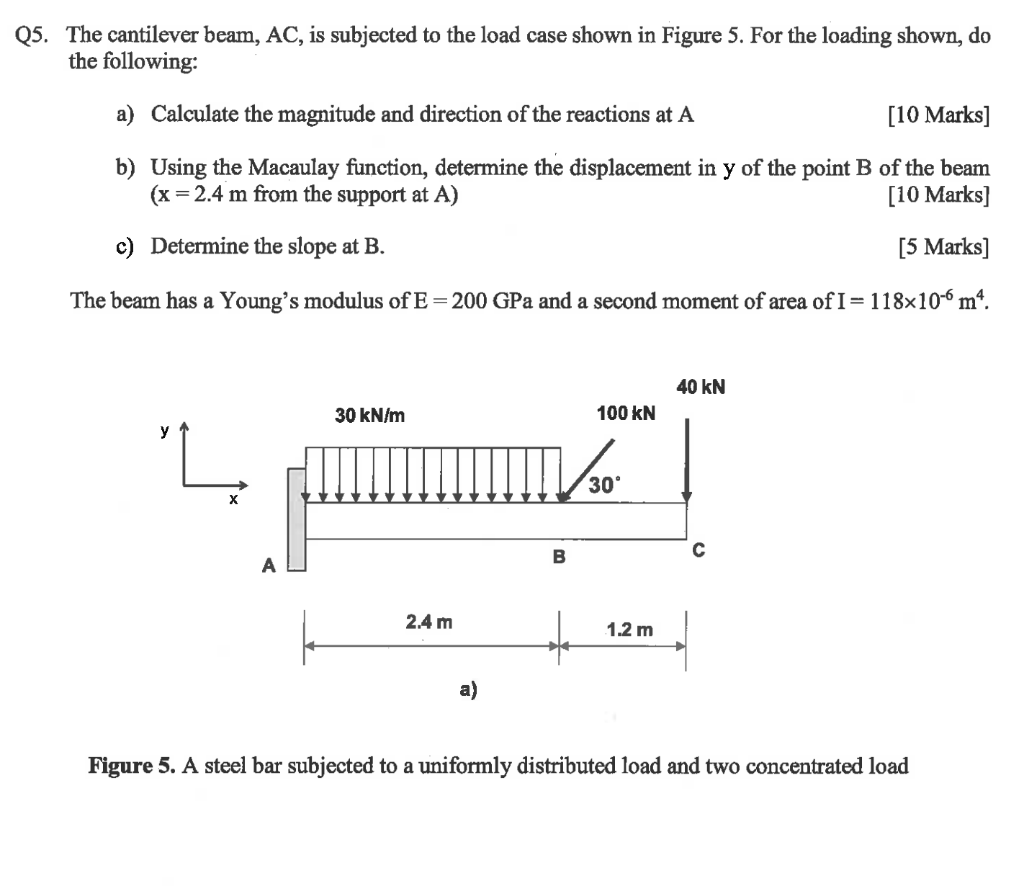Q5. The cantilever beam, AC, is subjected to the load case shown in Figure 5. For the loading shown, do the following: [10 Marks] a) Calculate the magnitude and direction of the reactions at A b) Using the Macaulay function, determine the displacement in y of the point B of the beam (x 2.4 m from the support at A) [10 Marks] c) Determine the slope at B. [5 Marks] The beam has a Young's modulus of E-200 GPa and...

• ### A Semi-infinite beam is loaded by force P at distance a from its end, as shown...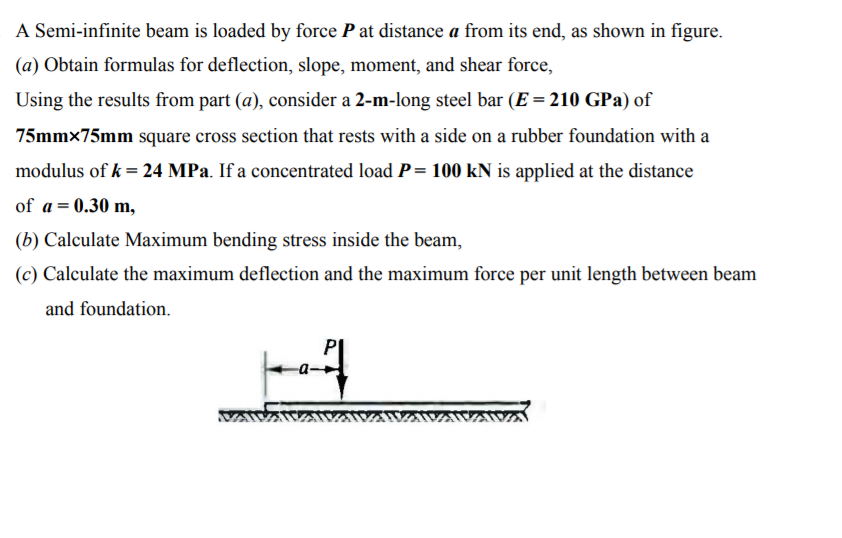A Semi-infinite beam is loaded by force P at distance a from its end, as shown in figure. (a) Obtain formulas for deflection, slope, moment, and shear force, Using the results from part (a), consider a 2-m-long steel bar (E = 210 GPa) of 75mm´75mm square cross section that rests with a side on a rubber foundation with a modulus of k = 24 MPa. If a concentrated load P = 100 kN is applied at the distance of a...

• ### Question 3 For the simply supported steel beam with cross section and loading shown (see Figure...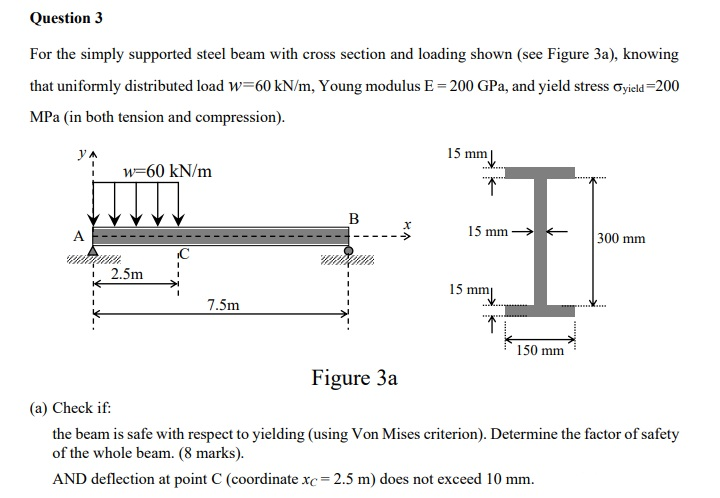Question 3 For the simply supported steel beam with cross section and loading shown (see Figure 3a), knowing that uniformly distributed load w=60 kN/m, Young modulus E = 200 GPa, and yield stress Cyield=200 MPa (in both tension and compression). ул 15 mm w=60 kN/m ... 1 B A 15 mm + 300 mm IC - i 2.5m 1 1 15 mm 7.5m 1 150 mm Figure 3a (a) Check if: the beam is safe with respect to yielding (using...